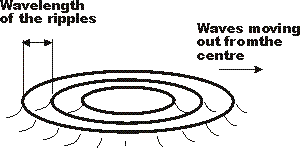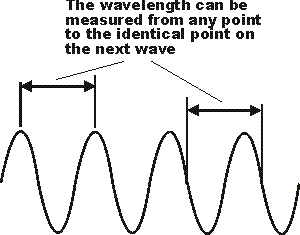## - an overview of what radio signals are and the relationship between frequency and wavelength

Radio signals are used for a huge variety of tasks. Radio signals are used to carry radio broadcasts, they are used to send signals to astronauts, establish wi-fi connections, for cellular communications and many, many more applications. Radio signals are essential to enable today's technology to function.

At the core of radio technology are the radio signals themselves. These radio waves have a variety of properties that we will investigate:

Radio signals or radio waves are a form of electromagnetic wave. Although this may sound complicated, it is possibly sufficient to say that these waves have both electric and magnetic components. They are the same as light rays, ultra-violet and infra-red. The only difference is in the wavelength of the waves.

To gain more of an idea of how the wave travels it can be likened to the surface of a pond when a stone is dropped into the water. Ripples spread out all around, decreasing in amplitude as they travel outwards. So it is with an electromagnetic wave although its action is somewhat more complicated.Radio signal analogy with ripples on a pond

## Frequency and wavelength

There are a number of properties of the radio wave that can be measured. One of the first to be measured was the wavelength. Radio stations originally used to have their position on a radio dial determined by the wavelength. For example the BBC used to have one of their transmitters broadcasting on a wavelength of 1500 metres. The wavelength of a radio wave is the distance between a point on one wave to the identical point on the next. One of the most obvious points to choose as a reference is the peak as this can be easily identified although any point is equally valid, provided the same point on each wave is taken.The wavelength of a radio signal

The frequency can be explained using the pond analogy. It is the number of times the wave goes up and down in a given time and at a particular point in the pond. The unit used for frequency is the Hertz and this corresponds to one cycle or wave per second. As frequencies which are encountered can be very high the standard prefixes of kilo (kiloHertz, kHz) for a thousand Hertz, Mega (MegaHertz, MHz) for a million Hertz, and Giga (GigaHertz, GHz) for a thousand million Hertz are commonly used.

## Speed of a radio signal

Another feature which can be noted about an electromagnetic wave is its speed. As it is the same as a light wave it has the same speed. Normally this is taken to be 3 x 10 8metres a second but a more exact figure is 299 792 500 metres a second in a vacuum.

## Wavelength and frequency conversion

The speed, frequency and wavelength of a radio wave are all related to one another. As the speed is virtually the same whether the signal is travelling in free space, or in the atmosphere, it is very easy to work out the wavelength of a signal if its frequency is known. Conversely the frequency can be calculated if the wavelength is known. The formula is very simply:

v = l x f

where
v =the velocity of the radio wave in metres per second (normally taken as 3 x 10 8m/s
l =the wavelength in metres
f =the frequency in Hertz

For example a signal with a frequency of 1 MHz will have a wavelength of 300 metres.